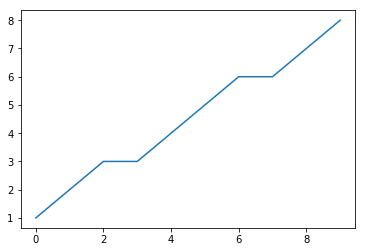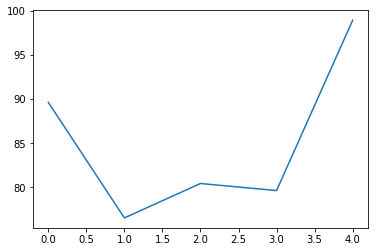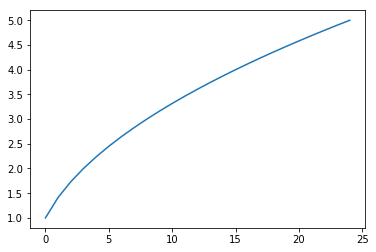## Modules Motivating Example

Throughout these tutorials, you may have noticed that Python seems to lack some fundamental operations. We learned that ** exponentiates a number, but what operation takes the square root? Python can find the minimum and maximum of a list, but how can we find the mean? Built-in Python doesn’t contain everything we need to perform all of the operations we want to perform. We can create functions and loops to replicate those missing operations, but recreating those functions every time we want to use them would be tedious and time-consuming. To solve this issue, we can use modules.

## Introduction to Python Modules

So far in our tutorials, we have been executing Python code from the terminal. All variables, objects, and output not saved to the disk will be erased after the terminal is closed. In this tutorial, we will explore the primary method of saving, using, and importing Python code between script executions. These saved Python scripts are called “modules,” which are used extensively in Python. Python modules not only save code for later execution, but are also used to import variables, functions, and class objects.

We mentioned in our Getting Started tutorial that Python modules are saved with the .py suffix. For example, we can save the following Python code with the name example.py:

print("The best of times")

Now we can execute this script from the command line terminal with the following:

python example.py
> The best of times

### Importing Python Modules & Objects

Python modules don’t only have to be executed as scripts: they can be used to import objects. There are a few methods we can use to import objects from a module, the simplest of which is the import [module] statement. For example, suppose we save the following code as example.py:

def addup(a, b):
return(a + b)

Now we can open a new script or terminal to import the module. When the module is imported into Python, its objects are referenced like object attributes with the module.object format. Now we can import the above example.py module and execute the addup function:

import example  # Notice that the .py suffix is implied
> 8

An alternative method for importing a module is the import [module] as [name] format, which will allow you to reference the module as [name] instead of its original name. This is useful for coding with modules that contain long or confusing names. For example:

import example as ex
> 8

If we wish to only import a selection of objects from a module, we can use the from [module] import [objects] format, where [objects] is a tuple of object names. If this method is used, only the named objects will be imported instead of the entire module. This effectively instantiates the objects within the current Python session, and allows the objects to be referenced directly. For example,

from example import addup
> 8

We can use a single asterisk * to tell Python to import all1 the objects contained within a module:

from example import *
> 8

Note: It is considered bad form to blindly import all objects from a module, as it can lead to variable naming collisions and take an unnecessary amount of memory. You should only use the import * statement if you are familiar with the module and its objects prior to use.

We can combine the selection import statement with the as statement to rename the objects:

from example import addup as AU
AU(5, 3)
> 8

I put together a Python Developer Kit with over 100 pre-built Python scripts covering data structures, Pandas, NumPy, Seaborn, machine learning, file processing, web scraping and a whole lot more - and I want you to have it for free. Enter your email address below and I'll send a copy your way.

Yes, I'll take a free Python Developer Kit

### Module Search Path, or "Where is my Module?!"

To beginners of Python, there is a very common and frustrating error that is associated with importing modules:

import numpy
> ModuleNotFoundError: No module named 'numpy'

As you may guess from the description, this exception is raised because Python cannot find the module you wish to import. Python searches for modules in the following order:

1. Python’s built-in modules
2. The directory in which Python is executed
3. Directories listed in the sys.path object

If the ModuleNotFoundError is raised, then Python could not find the module in these three locations. We can examine which directories are listed in the sys.path object by importing the built-in sys module and printing the sys.path list:

import sys
print(sys.path)
> [...list of path strings...]

I’ve omitted the actual list of path strings in the above example, as they vary between environments. If we want to add a directory for Python to check for modules, it’s as simple as appending a new string containing the path to the sys.path list:

sys.path.append([path string])

### dir Function

The dir function operates on modules similar to how it works on objects as we mentioned in the previous tutorial. The dir([module]) function will list the objects available for reference within the given module.

## Important Built-in Modules

Vanilla installations of Python come with several built-in modules that contain useful tools for basic operations. This tutorial won’t cover all the built-in modules, but we will cover a few of the basics. Python modules involved in file input and output will be discussed in a later tutorial devoted to input/output.

### math and statistics Modules

As you might guess, the math module provides a set of mathematical constants and functions, and the statistics module likewise provides statistical resources. For example:

import math
import statistics
math.pi  # Returns the constant pi
> 3.141592653589793
math.cos(0)  # Return the cosine of an input float
> 1.0
math.sqrt(4) # Return the square root of an input float
> 2.0
statistics.mean([1.0, 2.0, 3.0, 4.0, 5.0])
> 3.0

### os Module

The os module provides a set of functions for interacting with the operating system. The following is a list of some of the functions contained within the os module.

- os.chdir: Changes the working directory path
- os.rename: Renames a file
- os.listdir: Lists all directories and files within the working directory
- os.mkdir: Create a new directory
- os.remove: Deletes a file
- os.rmdir: Deletes a directory


Examples and further use of these functions will be expanded upon in a future tutorial.

### sys Module

The sys module contains functions for interacting with and changing the current instance of the Python interpreter. This is used to change the default behavior of Python, and to provide additional technical information. We have seen previously that this module contains the sys.path list that Python uses to find modules. The vast majority of the sys module is outside the scope of an introductory tutorial, however you should know what this module is and what it’s used for.

### random Module

The random module is used to generate pseudo-random numbers for the use of non-deterministic algorithms.

- random.seed: Initializes the pseudo-random number generator to a common state
- random.randrange: Returns a random integer from a given range
- random.shuffle: Shuffles a given sequence in place
- random.sample: Returns a sample of elements from a sequence
- random.uniform: Returns a random real-valued number from a given uniform distribution
- random.normalvariate: Returns a random real-valued number from a normal distribution


## Noteworthy Third-Party Modules

One of Python’s most powerful features is its support by the Python community. Thousands of Python modules are available for open-source usage that perform an endless number of functions. In this tutorial I’ll introduce a few of the most important and well known of these open-source modules. Each of these packages can take entire courses to master, therefore I’ll only perform a high-level review of each.

### scipy Module

The scipy module is available from SciPy.org. SciPy implements a library of numeric functions for scientific computing. These functions include methods for numeric integration, linear algebra, and other special functions. Most IDEs such as Anaconda will include SciPy and its collection of core packages, however it will need to be downloaded for use in vanilla Python.

### numpy Module

The numpy module is available from NumPy.org as a core package of SciPy. As we mentioned before in our tutorial on data structures, NumPy provides a data structure for implementing arrays. NumPy also provides a suite of mathematic operations. For example,

import numpy as np
l = [1, 2, 3]
a = np.array(l)
print(a)
> [1 2 3]
np.dot(a, a)  # Return the dot product of array a to itself
> 14

### matplotlib Module

The matplotlib module is available from the Matplotlib Page as another core package of SciPy. matplotlib is a toolbox for creating vector graphic plots. For example, we can create a simple plot using matplotlib sub-package pyplot with the following code:

import matplotlib.pyplot as plt
plt.plot([1,2,3,3,4,5,6,6,7,8])
plt.show()The matplotlib contains an enormous number of features for plotting, saving, and updating graphs. Further details on using matplotlib will be covered in later tutorials.

### pandas Module

The pandas module is available from the Pandas Page as another core package of SciPy. pandas contains functions and data structures used for data analysis. Similar to how numpy implements arrays, pandas implements data frames with associated operations. Pandas is an extensive package that is heavily used in the data science and analytics industry.

For example, we can define an random data frame for analysis:

import pandas as pd
import numpy as np
index = [1, 2, 3, 4]
colnames = ["Red", "Blue", "Green"]
df = pd.DataFrame(np.random.randn(4,3), index=index, columns=colnames)
print(df)
>         Red      Blue     Green
> 1  0.102140  0.519480 -0.039622
> 2  0.235870 -1.361429  0.618649
> 3 -1.383038 -0.260082  0.267085
> 4  0.440660  0.178628 -0.663830
df.describe()  # Pandas can perform fast summary statistics
>             Red      Blue     Green
> count  4.000000  4.000000  4.000000
> mean  -0.151092 -0.230851  0.045570
> std    0.833012  0.818480  0.544057
> min   -1.383038 -1.361429 -0.663830
> 25%   -0.269154 -0.535419 -0.195674
> 50%    0.169005 -0.040727  0.113731
> 75%    0.287067  0.263841  0.354976
> max    0.440660  0.519480  0.618649
print(df[df > 0])  # We can screen values based on Boolean expressions
>        Red      Blue     Green
> 1  0.10214  0.519480       NaN
> 2  0.23587       NaN  0.618649
> 3      NaN       NaN  0.267085
> 4  0.44066  0.178628       NaN

I put together a Python Developer Kit with over 100 pre-built Python scripts covering data structures, Pandas, NumPy, Seaborn, machine learning, file processing, web scraping and a whole lot more - and I want you to have it for free. Enter your email address below and I'll send a copy your way.

Yes, I'll take a free Python Developer Kit

## Python Modules Example

Lets look back at our previous geography teacher example that we used in the tutorial on Class Objects. In the previous tutorial we defined a class object geographyStudent using the following code:

geographyClass = [{"Name":"Mary" ,"HW":85 ,"P1":90 ,"M":88 ,"P2":100 ,"F":90},
{"Name":"Matthew" ,"HW":80 ,"P1":95 ,"M":70 ,"P2":90 ,"F":70},
{"Name":"Marie" ,"HW":90 ,"P1":92 ,"M":84 ,"P2":0 ,"F":91},
{"Name":"Manuel" ,"HW":79 ,"P1":70 ,"M":85 ,"P2":70 ,"F":82},
{"Name":"Malala" ,"HW":100 ,"P1":95 ,"M":100 ,"P2":98 ,"F":99}]
class geographyStudent:
def __init__(self, input):  #Initializing each grade attribute
self.name = input["Name"]
self.homework = input["HW"]
self.project1 = input["P1"]
self.midterm = input["M"]
self.project2 = input["P2"]
self.final = input["F"]
+  self.project1*0.1
+  self.midterm*0.2
+  self.project2*0.1
+  self.final*0.4)
students = []
for s in geographyClass:
students.append(geographyStudent(s))

This was a somewhat bulky piece of code, and if we had hundreds of students then we would have to copy a significant amount of code each time we wanted to perform an analysis. Let’s save the above code to a module called students.py. Now, we can import and use the student objects:

from students import students  # Import the students list from the students module
students.name
> Mary

Now we can use the statistics package to compute some statistics of the students’ grades:

from students import students
import statistics as stats
> 85.0
> 9.17796273690409

We can also use the matplotlib to plot the grades:

from students import students
import matplotlib.pyplot as plt
plt.show()## Python Modules Practice Problems

1. Use the math module to determine the hyperbolic cosine of $2\pi$. (Hint: Use the Python Module Index to look up these functions and constants.)     Solution

2. Use the math and matplotlib modules to plot the square roots of the numbers from 1 to 25.     Solution

3. Suppose you are working on a new coding project, and you wish to use a set of functions you wrote in the module toolbox.py located in the old project directory C:\\Users\\Me\\OldProject. Attempting to import the module using import toolbox raises a ModuleNotFoundError exception. What is an efficient way to correct this error?     Solution

4. Create a random anagram of the of the string laksdoimpoaiflkasdufpoiemfoeiwjsdlkfjgliuajrliulihrlia. (Hint: Use the shuffle function of the random module)     Solution

5. Convert the number 4.265 to a fraction using the fraction module. (Hint: Use the Python Module Index to look up these functions.)     Solution

## Solutions

1. This can be performed with the following:

import math
print(math.cosh(2*math.pi))
> 267.7467614837482

2. This can be performed with the following:

import math
import matplotlib.pyplot as plt
x = [math.sqrt(i) for i in range(1, 26)]
plt.plot(x)
plt.show()3. Recall the issue here is that Python cannot find the toolbox.py module in either the the built-in modules, the working directory, or the sys.path listing. We can eliminate the error by adding the directory of the file to the sys.path listing:

import sys
sys.path.append("C:\\Users\\Me\\OldProject")
import toolbox

4. We can apply the shuffle function to the string by first transforming it into a list of characters:

import random
a = list("laksdoimpoaiflkasdufpoiemfoeiwjsdlkfjgliuajrliulihrlia")
random.shuffle(a) # a is now a shuffled list of characters
a = "".join(a)  # This will concatenate the list of characters into a new string
print(a)  # Output omitted as it will change between sessions

5. To solve this problem, you will need to lookup the documentation for the fraction module and understand its functionality. We can convert the number to a fraction using the Fraction object:

from fractions import Fraction
Fraction(4.265).limit_denominator()
> Fraction(853, 200)

1. Recall from the tutorial on Python class objects that any object prefixed with a single leading underscore (_atr) is considered “private”, and will not be imported.